Question

3. Draw the region D and evaluate the double integral using polar coordinates. dA, D= {(x,...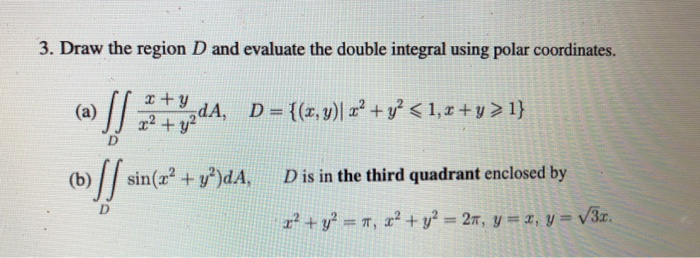3. Draw the region D and evaluate the double integral using polar coordinates. dA, D= {(x, y)| x2 + y² <1, x +y > 1} (b) sin(x2 + y2)dA, D is in the third quadrant enclosed by D r? + y2 = 7, x² + y2 = 24, y = 1, y = V3r.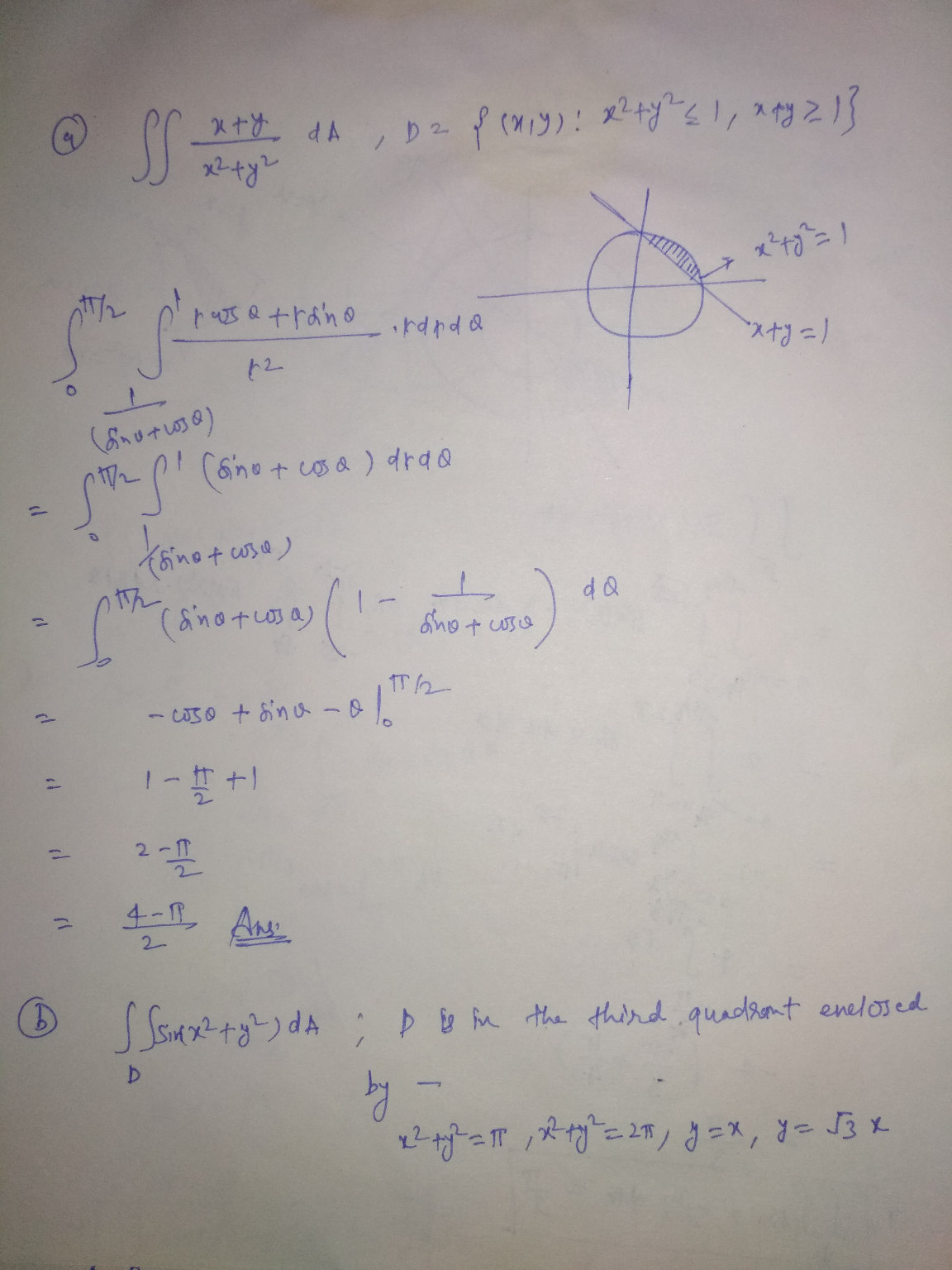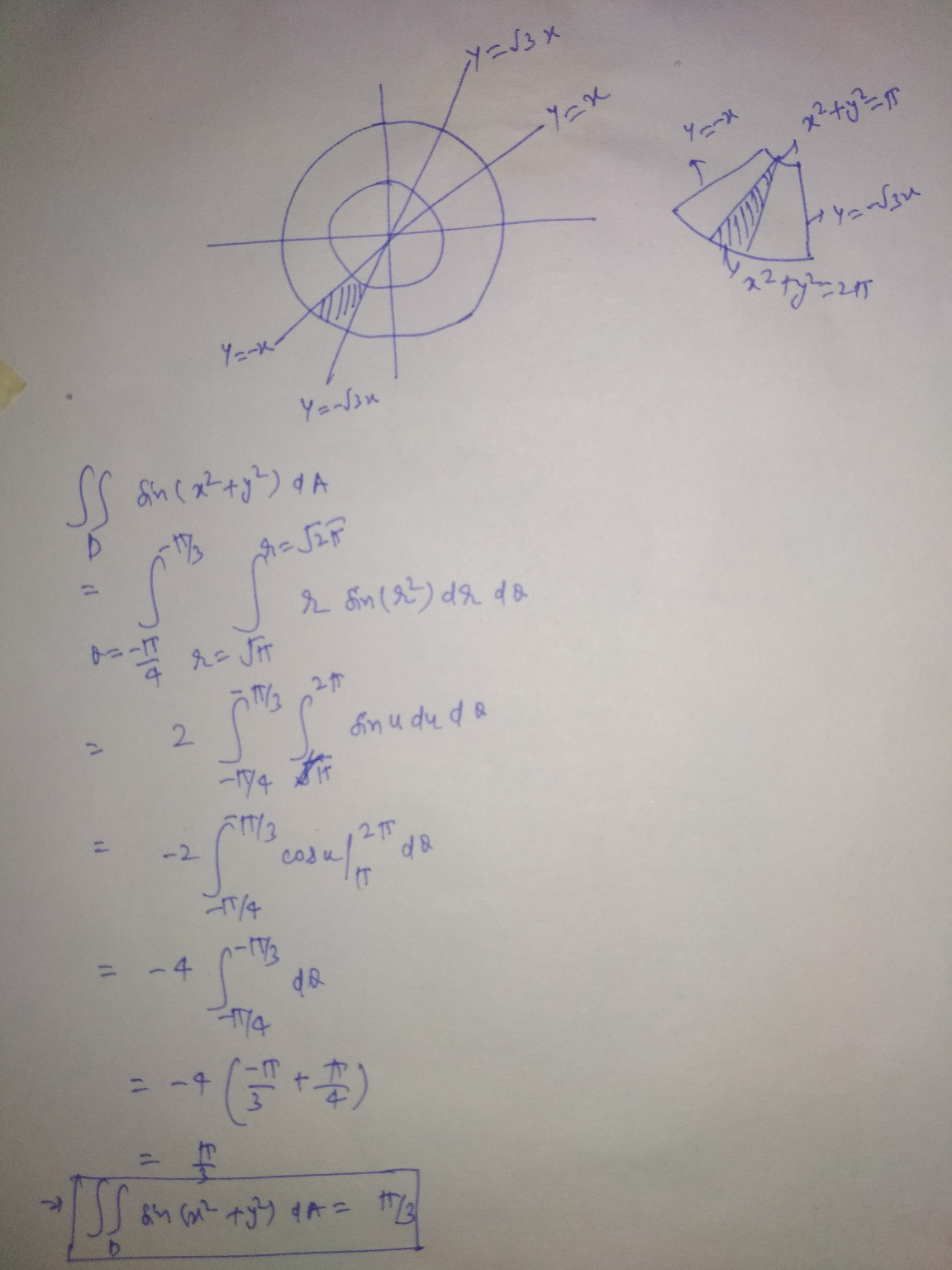Earn Coins

Coins can be redeemed for fabulous gifts.

Similar Homework Help Questions
• 3. Draw the region D and evaluate the double integral using polar coordinates. (a) SI x...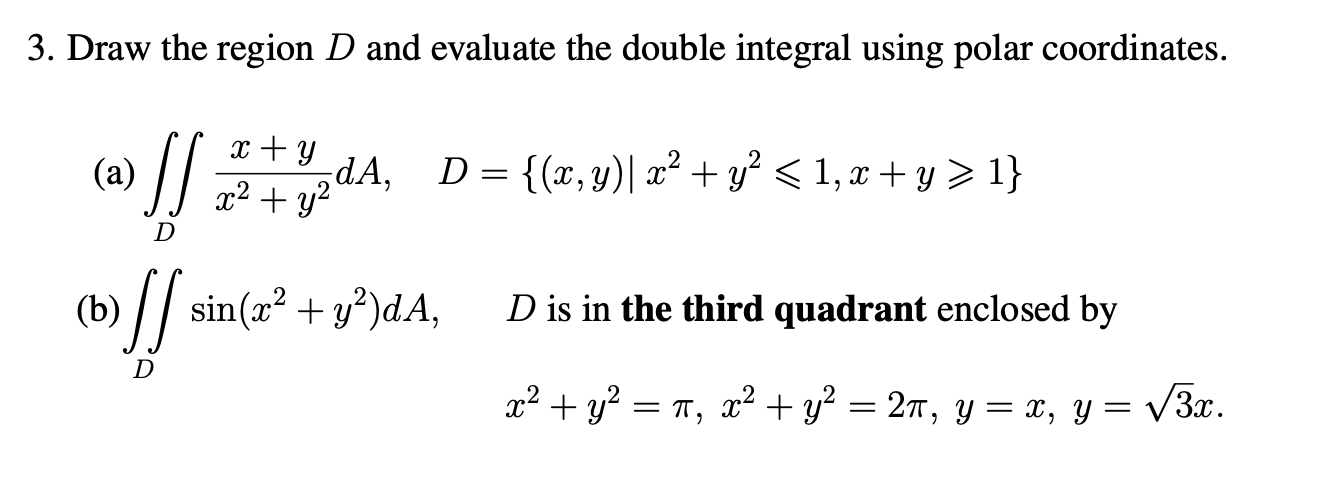3. Draw the region D and evaluate the double integral using polar coordinates. (a) SI x + y dA, x2 + y2 D= {(x, y)| x2 + y2 < 1, x + y > 1} D (b) ſ sin(x2 + y2)dA, D is in the third quadrant enclosed by m2 + y2 = 71, x2 + y2 = 27, y=x, y= V3x.

• (1 point) Using polar coordinates, evaluate the integral ST sin(x2 + x>)dA where Ris the region...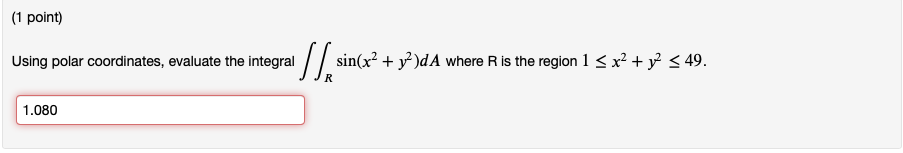(1 point) Using polar coordinates, evaluate the integral ST sin(x2 + x>)dA where Ris the region 1 5x2 + y2 549. 1.080

• 5.Use polar coordinates system to evaluate: x2 + y2)dydx , R is the region enclosed by...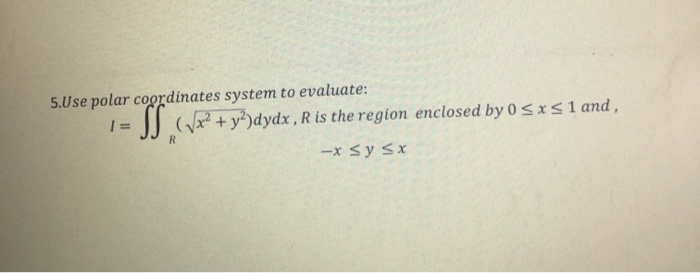5.Use polar coordinates system to evaluate: x2 + y2)dydx , R is the region enclosed by 0 <x< 1 and, -x sy sx

• Evaluate the given integral by changing to polar coordinates. R (4x − y) dA, where R...

Evaluate the given integral by changing to polar coordinates. R (4x − y) dA, where R is the region in the first quadrant enclosed by the circle x2 + y2 = 4 and the lines x = 0 and y = x

• 1. Use polar coordinates to evaluate the double integral dA z2 +y where R is the...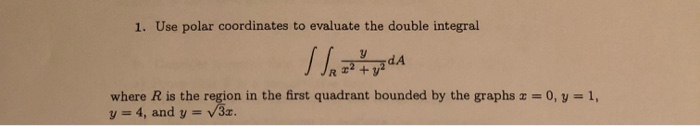1. Use polar coordinates to evaluate the double integral dA z2 +y where R is the region in the first quadrant bounded by the graphs x = 0, y = 1, y=4, and y V3z. 1. Use polar coordinates to evaluate the double integral dA z2 +y where R is the region in the first quadrant bounded by the graphs x = 0, y = 1, y=4, and y V3z.

• 3. Evaluate the integral by changing to polar coordinates: SS (x+y) da R Where R is...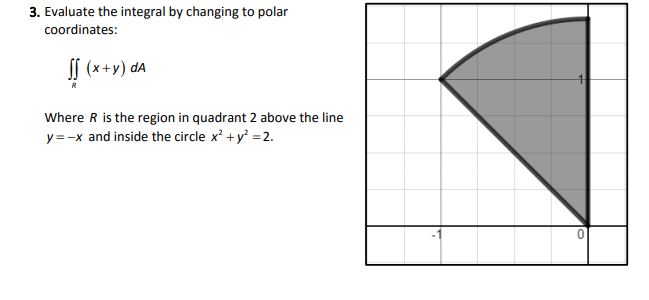3. Evaluate the integral by changing to polar coordinates: SS (x+y) da R Where R is the region in quadrant 2 above the line y=-x and inside the circle x2 + y2 = 2.

• polar coordinates double integrals

Evaluate the given integral by changing to polar coordinates.The integral isdouble int (x)dAwhere D is the region in the first quadrant that lies between the circles x2 + y2 = 64 and x2 + y2 = 8x.

• 3. Let D be the region in the first quadrant lying inside the disk x2 +y2...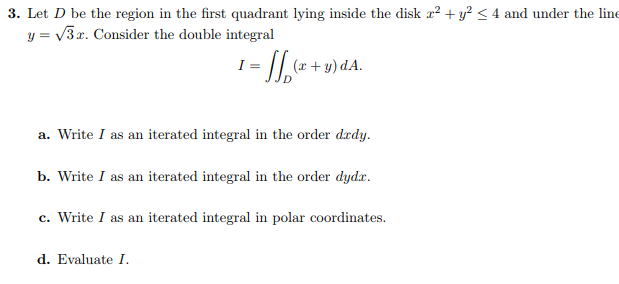3. Let D be the region in the first quadrant lying inside the disk x2 +y2 < 4 and under the line y-v 3 x. Consider the double integral I-( y) dA. a. Write I as an iterated integral in the order drdy. b. Write I as an iterated integral in the order dydx c. Write I as an iterated integral in polar coordinates. d. Evaluate I

• 7. Evaluate the following integral by converting to polar coordinates: S], 127 (2x – y)dA, where...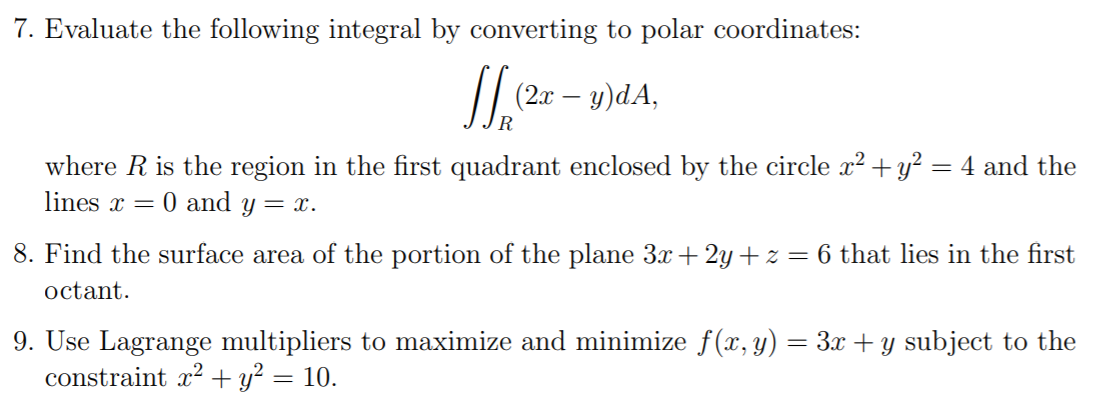7. Evaluate the following integral by converting to polar coordinates: S], 127 (2x – y)dA, where R is the region in the first quadrant enclosed by the circle x2 + y2 = 4 and the lines x = 0 and y = x. 8. Find the surface area of the portion of the plane 3x + 2y +z = 6 that lies in the first octant. 9. Use Lagrange multipliers to maximize and minimize f(x, y) = 3x + y...

• Use polar coordinates to evaluate the integral where R is the region in in the first...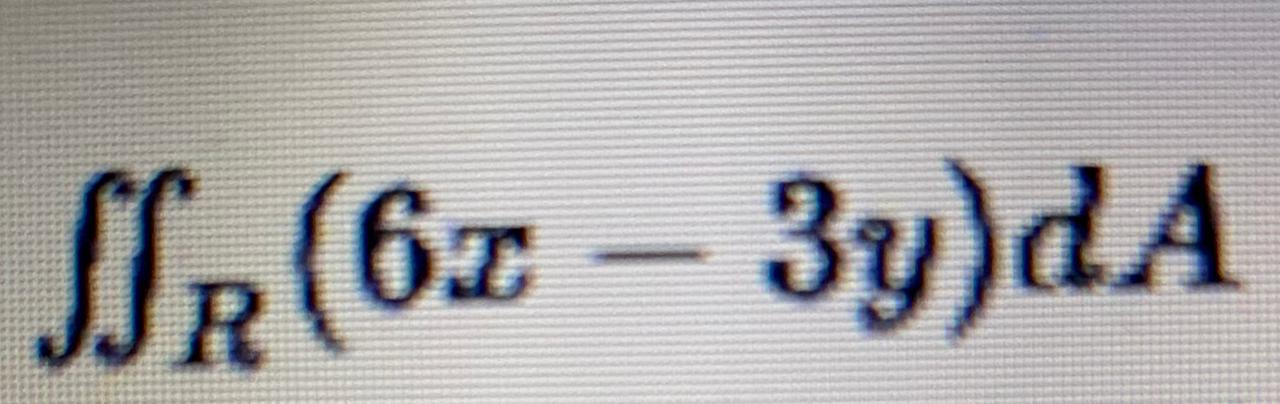Use polar coordinates to evaluate the integral where R is the region in in the first quadrant enclosed by the circumference x2+y2=4 and the lines x=0 and y=x SUR (60 - 3y)dA Use coordenadas polares para evaluar la integral JR (6x – 3y)dA donde R es la región en en el primer cuadrante encerrada por la circunferencia za + y2 = 4y las rectas r = Oyy=2. 0-8+12V2 O NO ESTÁ LA RESPUESTA O 16 - 12/2 O 12 -...